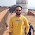# EE1203 Electrical Machines Laboratory – I Syllabus

EE 1203 ELECTRICAL MACHINES LABORATORY – I 0 0 3 100

AIM
To expose the students to the operation of D.C. machines and transformers and give them experimental skill.

1. Open circuit and load characteristics of D.C separately and self excited shunt generator

2. Load characteristics of D.C. compound generator with differential and cumulative connection

3. Load characteristics of D.C. shunt and compound motor

5. Load characteristics of D.C series motor

6. Swinburne’s test and speed control of D.C
shunt motor

7. Hopkinson’s test on D.C motor – generator set

7. Load test on single-phase transformer and three phase transformer connections

8. Open circuit and short circuit tests on single phase transformer

9. Sumpner’s test on transformers

10. Separation of no-load losses in single phase transformer

P = 45 Total = 45

Detailed Syllabus

1. Open Circuit and Load Characteristics of DC Separately and self excited shunt Generator

Aim
To conduct no load and load test on self and separately excited generators and obtain the characteristics.
Exercise
1. Obtain the open circuit characteristics of a separately and self excited D.C generator and determine critical resistance.

2. Draw the external and internal characteristics of a separately and self excited D.C generator and compute full load regulation.

2. Load Characteristics Of D.C. Compound Generator with differential and cumulative connection
Aim
To conduct load test on DC compound generator and obtain the load characteristic curves
Exercise
1. Obtain the following curves for cumulative, differential and shunt generator

a. IL Vs V for DC cumulative compound generator
b. IL Vs V for DC differential compound generator

All graphs should be drawn on the same graph sheet

3. Load characteristics of DC Shunt and compound motor
Aim
To conduct load test on DC shunt motor and compound motor and draw the
characteristic curves

Exercise
1. Draw the following characteristic curves for DC shunt and compound motor

a. Output Vs η%
b. Output Vs T
c. Output Vs N
d. Output Vs IL
e. Torque Vs N

4. Load characteristics of DC series motor
Aim
To conduct load test on DC series motor and draw the characteristics curves

Exercise

1. Draw the following characteristics curve for DC series motor

a. Output Vs η%
b. Output Vs T
c. Output Vs N
d. Output Vs I
e. Torque Vs N

5. Swinburne’s Test and speed control of DC shunt motor
Aim
To conduct Swinburne’s test and predetermine the performance characteristics of DC machine and speed control of DC motor
Exercise

1. Predetermine efficiency at various load current while operating as a motor and generator and plot a graph output Vs η%

2. Draw the following curves for

a. If Vs N at Va = 0.8 Va and Va
b. Va Vs N at 0.8 If and If

6. Hopkinson’s Test on DC motor – Generator set
Aim
To conduct Hopkinson’s test on a pair of DC shunt machines and determine their
efficiency.

Exercise
1. Determine the stray losses of the machines.
2. Obtain efficiency curves for the motor and generator and draw the curves.

7. Load Test On Single-Phase Transformer and three phase transformer connections
Aim
To conduct load test on the given single phase transformer and determine its
Performance.

Exercise
1. Draw the following graph for single phase transformer
a. Output Vs η%
2. To carry out the following three phase transformer connection
Y-Y; Y-Δ; Δ – Δ; Δ – Y
Check the input output voltage ratio for various three phase connection.

8. Open Circuit and Short Circuit Tests on Single Phase Transformer
Aim
To conduct O.C and S.C test on a single phase transformer and calculate the
performances

Exercise

1. Determine the equivalent circuit of the transformer.
2. Predetermine the efficiency at different load at UPF and 0.8 Power factor lagging.
3. Predetermine the full load regulation at different power factor.
4. Draw the following curves
a. Output Vs η%
b. Power factor Vs %Regulation

9. Sumpner’s Test on transformers
Aim
To conduct Sumpner’s test on a pair of identical single phase transformers and
predetermine performance.

Exercise
1. Study the paralleling process for two identical transformers.

2. Determine the equivalent circuit parameters of each transformer.

3. Predetermine the efficiency at different loads at 0.8 and 1.0 power factors.

4. To predetermine the full load regulation for different power factors.

5. Draw the following graph

a. Output Vs %η
b. Power factor Vs %Regulation

10. Separation of No-Load Losses in Single Phase Transformer
Aim
To separate the iron losses of a single phase transformer into its components
a. Hysterisis losses
b. Eddy current losses
Exercise
1. Separate the no load losses into hysterisis and eddy current components.

Previous
Next Post »